Welcome to class!

In today’s class, we will be talking about radioactivity. Enjoy the class!Radioactivity was discovered by Henri Becquerel in 1869. In 1898, Marie and Pierre Curie succeeded in chemically isolating two radioactive elements, Polonium (z=84) and Radium (z= 88).

Radioactivity or radioactive decay is the spontaneous disintegration of unstable nuclides to form stable ones with the emission of radiation.

Unstable nuclides continue to disintegrate until a stable atom is formed.

Alpha () and beta () particles are emitted and the gamma rays () accompany the ejection of both alpha and beta particles.

#### The nucleus

The nucleus is made up of protons and neutrons.

They are surrounded by negatively charged ions known as electrons.

The number of protons is equal to the number of electrons. Both protons and neutrons have the same mass.

The weight of an electron is relatively small compared to neutrons and protons.

The number of protons in an atom is referred to as the proton number (atomic number) and denoted by the symbol Z.

the number of neutrons is denoted by the symbol N. Protons and neutrons are called nucleons since they form the nucleus of an atom.

The sum of both the protons and neutrons is called the mass number A or nucleon number.

Therefore;

A = Z + N and N = A – Z.

The masses of atoms are conveniently given in terms of atomic mass units (ᴜ) where (ᴜ) is 1/12th the mass of one atom of carbon-12 and has a value of 1.660 × 10-27 kg.

Hence the mass of one proton is equal to 1.67 × 10-27 and is equal to 1ᴜ.

Isotopes are elements with different mass numbers but with equal atomic numbers i.e. uranium with mass numbers 235 and 238.

An alpha particle is a helium nucleus 42He. Whenever a nucleus goes through alpha decay, it transforms into a different nucleus by emitting an alpha particle. For example, when 23892U undergoes alpha-decay, it transforms into 23490Th.

23892U → 23490Th + 42He … (1)

Now, 42He contains two protons and two neutrons. Hence, after emission, the mass number of the emitting nucleus reduces by four and the atomic number reduces by two. Therefore, the transformation of AZX nucleus to A-4Z-2X nucleus is expressed as follows,

AZX → A-4Z-2X + 42He … (2)

Where AZX is the parent nucleus and A-4Z-2X is the daughter nucleus. It is important to note that the alpha decay of 23892U can occur without an external source of energy. This is because of the total mass of the decay products (23490Th and 42He) < the mass of the original 23892U

Or, the total mass-energy of the decay products is less than that of the original nuclide. This brings us to the concept of ‘Q value of the process’ or Disintegration energy’ which is the difference between the initial and final mass-energy of the decay products.

This energy is shared between the daughter nucleus, A-4Z-2X and the alpha particle, 42He in the form of kinetic energy. Also, alpha decay obeys radioactive laws.

##### Alpha decay

A nucleus emitting an alpha particle reduces its mass by 4 atomic mass units and its proton number by 2. The equation can be written as follows,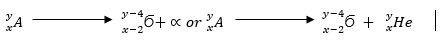Properties

1. Their speeds are 1.67 × 107 m/s, which is 10% the speed of light.
2. They are positively charged with a magnitude of a charge double that of an electron.
3. They can be affected by photographic plates and produce flashes when

Worked examples

(1) Uranium 235  changes to Thorium by emitting an alpha particle. Write a number equation to represent the decay

SolutionThe change of an element (nucleus) to another is called transmutation.

Beta-decay is when a nucleus decays spontaneously by emitting an electron or a positron. This is also a spontaneous process, like the alpha decay, with definite disintegration energy and half-life. And, it follows the radioactive laws. Beta-decay can be a beta minus or beta plus decay.

In a Beta minus (β) decay, an electron is emitted by the nucleus. For example,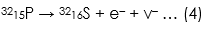where v- is an antineutrino, a neutral particle with little or no mass. Also, T1/2 = 14.3 days.

n a Beta plus decay, a positron is emitted by the nucleus. For example,where v is a neutrino, a neutral particle with little or no mass. Also, T1/2 = 2.6 years.

Also, in a Beta plus decay, a proton transforms into a neutron (inside the nucleus):

Hence, we can see that the mass number (A) of the emitting nuclide does not change. As shown in equations (6) and (7), either a proton transforms into a neutron or vice versa.

(b) Beta (ϐ) particles

They are represented by meaning that they have no mass but a charge of -1. Properties

1. Their speeds are as high as 99.9% or more the speed of light
2. They are deflected by electric and magnetic fields but in a direction opposite to that of alpha particles.
3. They can be stopped by a thin sheet of aluminium
4. Their ionization power is much less intense about 1/100th that of alpha particles.
##### Beta-decay

The beta particle is an electron. Beta particles are produced by changing a neutron to a proton and later to an electron as shown,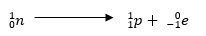The electron is then ejected from the nucleus and the number of protons increases by 1 while the mass number remains the same (an electron is of negligible mass).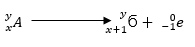Worked examples

(1) Thorium changes to Proctanium (P0) with the emission of a beta particle. Show the decay using the nuclear equation.

Solution(2) Write an equation to show how a radioactive isotope of cobalt (o) undergoes a beta decay followed by the emission of gamma rays to form a new nuclide X.

Solution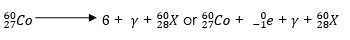(3) A radioactive carbon – 14 decays to nitrogen by emitting a beta particle as shown.

Determine the values of ‘x’ and ‘y’ in the equation below.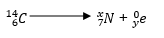Solution

X + 0 =14 hence x = 14

7 + y = 6 hence y = – 1

We know that atoms have energy levels. Similarly, a nucleus has energy levels too. When a nucleus is in an excited state, it can transition to a lower energy state by emitting electromagnetic radiation. Further, the difference between the energy states in a nucleus is in MeV. Hence, the photons emitted by the nuclei have MeV energies and called Gamma rays.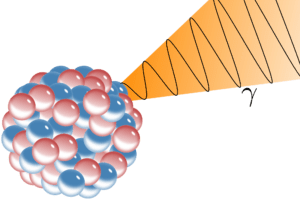After an alpha or beta emission, most radionuclides leave the daughter nucleus in an excited state. This daughter nucleus reaches the ground state by emitting one or multiple gamma rays. For example,

6027Co undergoes beta decay and transforms into 6028Ni. The daughter nucleus (6028Ni) is in its excited state. This exciting nucleus reaches the ground state by the emission of two gamma rays having energies of 1.17 MeV and 1.33 MeV. The energy level diagram shown below depicts this process.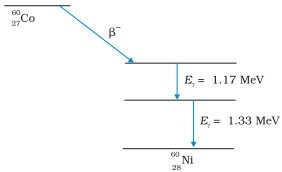##### Gamma particles

They have very short wavelengths in the order of 10-10 m and below.

Properties

1. They travel at the speed of light.
2. They have less ionization power than that of both alpha and beta particles
3. They accompany the emission of alpha and beta particles
4. They have more penetrating power than X-rays.

(i) Medical use

Many diseases such as cancer, leukaemia etc., are cured by radiation therapy.

(ii) Scientific use

Radioactive tracers are used in agricultural science to study the growth of plants.

(iii) Industrial use

Radioisotopes are used as fuel for atomic energy reactors.

###### General evaluation

Assignment

(1)In our next class, we will be talking about the Transformation of Elements. We hope you enjoyed the class.

Should you have any further question, feel free to ask in the comment section below and trust us to respond as soon as possible.

How Can We Make ClassNotesNG Better - CLICK to Tell Us💃